# C语言中的循环语句

for循环语句的格式如下:
for(初始化部分;运行条件检查部分;条件变量变化部分){
循环体;
}

...

for(i=1,sum=0;i<=10;i++){
sum+= i;
}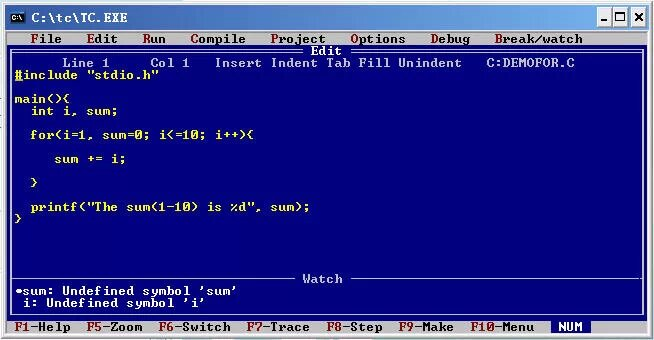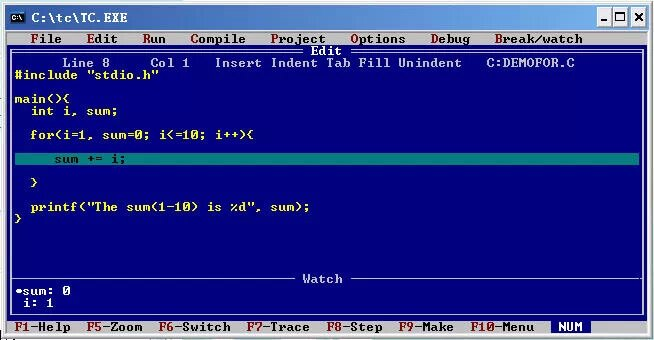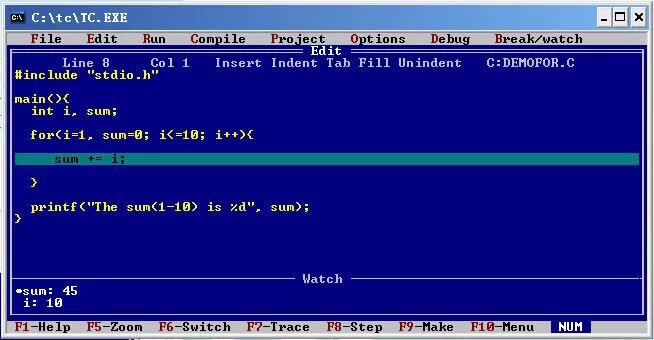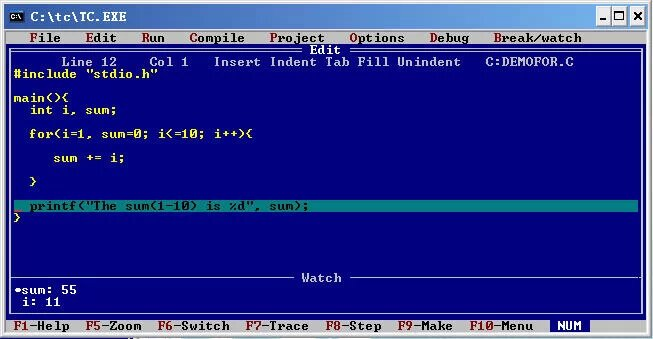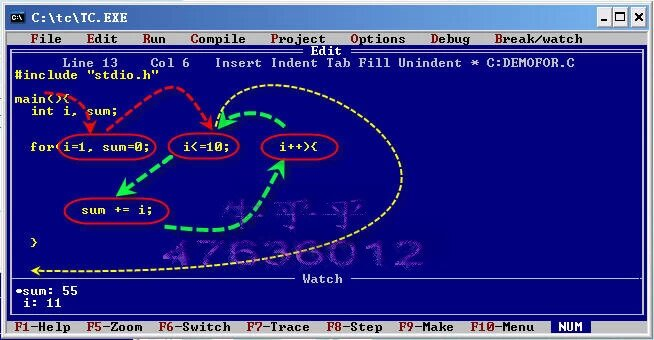step1:先初始化
step2:判断是否满足循环条件,满足由绿线进入step3;不满足由黄线跳出循环体step5
step3:执行循环体
step4:改变循变量,go step2
step5:结束循环

for(i=1,t=1;  ;i++){
t*=i;
if (t>=200) break;
}/*此循环是break跳出循环,这时候可以把循环判断条件部分省略.我们也可以看出,一个循环中可以有多个出口,即多个退出循环的地方.值得注意的是,必须知道每个地方在什么情况下跳出来:)*/

i=1;
sum=0;
for(;i<10;i++){
sum += i*i;
}

for(;;){
}

------------------------

i=0;
sum=0;
while(sum<1000){
sum+=i;
i++;
}

while 循环应用的地方也很多,但是和for语句不同的是,我们经常用for控制循环次数比较明显的地方.while的优势是集中精力与处理条件循环.

i=0;
sum=0;
do{
sum += i*i;
i++;
}while(i<10);

i=10;
sum=0;
do{
sum+=i*i;
}while(i<10);

-----
i=10;
sum=0;
while(i<10){
sum+=i*i;
}

https://wap.sciencenet.cn/blog-645848-1179539.html

## 相关博文

GMT+8, 2021-8-4 16:46